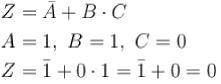Courses

# Digital Electronics NAT Level - 1

## 10 Questions MCQ Test Solid State Physics, Devices & Electronics | Digital Electronics NAT Level - 1

Description
This mock test of Digital Electronics NAT Level - 1 for IIT JAM helps you for every IIT JAM entrance exam. This contains 10 Multiple Choice Questions for IIT JAM Digital Electronics NAT Level - 1 (mcq) to study with solutions a complete question bank. The solved questions answers in this Digital Electronics NAT Level - 1 quiz give you a good mix of easy questions and tough questions. IIT JAM students definitely take this Digital Electronics NAT Level - 1 exercise for a better result in the exam. You can find other Digital Electronics NAT Level - 1 extra questions, long questions & short questions for IIT JAM on EduRev as well by searching above.
*Answer can only contain numeric values
QUESTION: 1

### If the memory chip size is 256 x 1 bits, what is the number of chips required to make 1 up 1K byte of memory

Solution:

1 byte = 8 bits
1K byte = 1024 bits
1K byte = 1024 x 8 bit
Number of chips =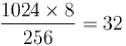*Answer can only contain numeric values
QUESTION: 2

### The number 6.25 in the decimal notation, when converted to the binary notation will read as ?

Solution: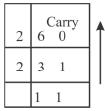Hence, 6 =110
Now 0.25 x 2 = 0.50 → 0
0.50 x 2 = 1.00 → 1
0.00 x 2 = 0 → 0
Hence, 0.25 = 0.010 →1
Hence (6.25)2 = 110.010

*Answer can only contain numeric values
QUESTION: 3

### What must be the output if A = 1 , B = 0, C = 1 ?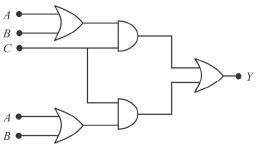Solution: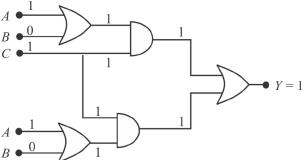Hence, output = 1

*Answer can only contain numeric values
QUESTION: 4

In the given circuit, if the inputs A = B = 0, then what is the output of D ?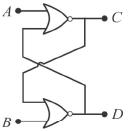Solution:

A = B = 0
Logic states of C and D will be 0, 0

*Answer can only contain numeric values
QUESTION: 5

The function F generated by the logic network shown gives what output for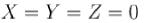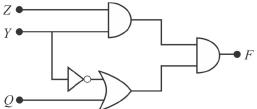Solution: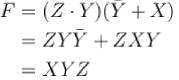Hence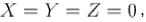gives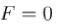*Answer can only contain numeric values
QUESTION: 6

The Boolean expression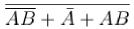is equivalent to ?

Solution: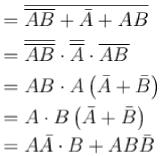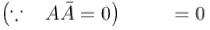*Answer can only contain numeric values
QUESTION: 7

The below logic circuit gives what output, if V1 = 0, V2 = 1 ?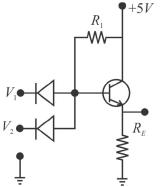Solution:

The above circuit behaves as a NAND gate.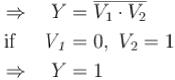*Answer can only contain numeric values
QUESTION: 8

If two input terminals of NAND gate are shorted, then the output for both the input being zero is ?

Solution: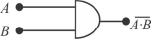If A and B are joined, then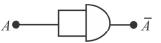This behaves like a NOT gate.

*Answer can only contain numeric values
QUESTION: 9

An OPAMP is connected in a circuit with a Zener diode as shown in the figure. The value of resistance R in kfl for obtaining a regulated output Vo = 9 V is: (Specify your answer to two digits after the decimal point.)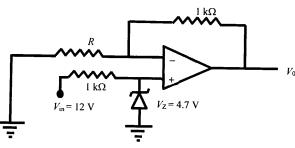Solution:*Answer can only contain numeric values
QUESTION: 10

For the Boolean expression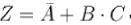what will  output for A= 1, B = 1, C = 0 ?

Solution: